### Genetic Algorithms for permutation problems (GAPerm)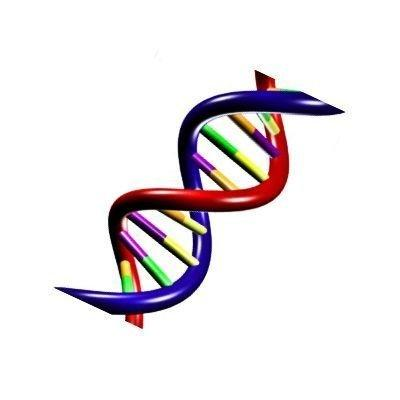## Introduction

xlOptimizer implements many variants of Genetic Algorithms [1for permutation problems. These are included in a solver named GAPerm. The permutation problems naturally take the form of deciding on the order in which a sequence of events should occur; for these problems, a natural representation is a permutation of a set of integers. There are two sub-cases of problems; the first includes problems in which the absolute order is important (such as the Job Shop Scheduling problem) while in the second only adjacency is important (a typical example is the Traveling Salesman Problem - TSP.

## How does it work?

The algorithm mimics the process of natural selection. A population of candidate solutions is evolved, generation by generation, using techniques inspired by natural evolution such as selection, mutation and crossover

The evolution starts from a population of randomly generated individuals. In each generation, the fitness of every individual in the population is evaluated. This is a measure of the quality of the solution, and it is depended on the objective function value corresponding to the solution. Next, multiple individuals are stochastically selected from the current population (according to their fitness) to become parents. Their genetic material is recombined, and possibly randomly mutated, to produce offspring and form a new population. The new population is then used in the next iteration of the algorithm. Commonly, the algorithm terminates when either a maximum number of generations has been produced, or a satisfactory fitness level has been reached for the elite (best) member of the population.

## Mathematical formulation

#### Pseudo-code

The GAPerm algorithm can be described by the following pseudo-code:

1. Initialize randomly the population of individuals (chromosomes).
2. Calculate the fitness of each individual in the population.
3. Select individuals to form a new population according to each one’s fitness.
4. Perform crossover and mutation to form a new population.
5. Copy the best (elite) individual to the new population.
6. Repeat steps (2–5) until some condition is satisfied.

#### Encoding

The GAPerm so uses permutations of integers, namely from 1 to D, where D is the number of design variables.

#### Fitness

The fitness of each chromosome is determined by the corresponding objective function value. This objective function is determined by the user. Usually it returns the cost of the candidate solution, and thus it is referred to as the cost function (to be minimized).

#### Initialization

Initially, solutions are randomly generated to form an initial population. The population size depends on the nature of the problem.

#### Selection

During each successive generation, a proportion of the existing population is selected to breed a new generation. Individual solutions are selected through a fitness-based process, where fitter solutions (as measured by a fitness function) are typically more likely to be selected. Certain selection methods rate the fitness of each solution and preferentially select the best solutions.

#### Reproduction: Crossover and mutation

The next step is to generate a new population of solutions using genetic operators: crossover (also called recombination), and/or mutation.

For each new solution to be produced, a pair of "parent" solutions is selected for breeding. Mutation is also stochastically applied to the offspring, which allows the GA to avoid entrapment into local optima and explore new regions of the search space. New parents are selected for each new child, and the process continues until a new population of solutions of appropriate size is generated.

#### Elitism

Elitism is used in order to ensure that (at least) the best individual of the current generation survives to the next one. Typically one individual, i.e. the best, is transferred as-is to the next generation.

#### Termination

This generational process is repeated until a termination condition has been reached. Common terminating conditions are:

• A solution is found that satisfies some criteria
• An allocated computation budget is reached
• The highest ranking solution's fitness is reaching or has reached a plateau such that successive iterations no longer produce better results
• Combinations of the above

## Options in xlOptimizer

The following options are available in xlOptimizer:

#### Step 1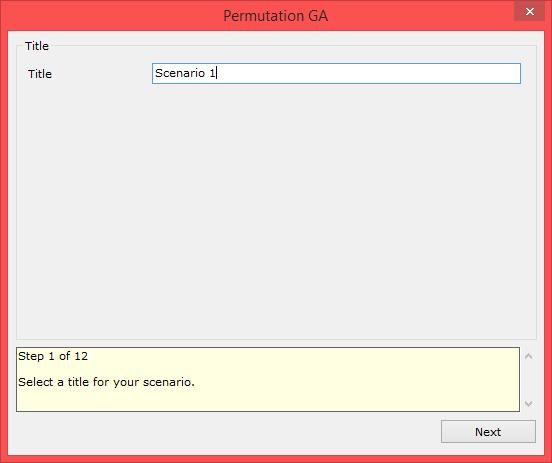Enter a short title for the scenario. The log files will be saved to a subfolder with this name.

#### Step 2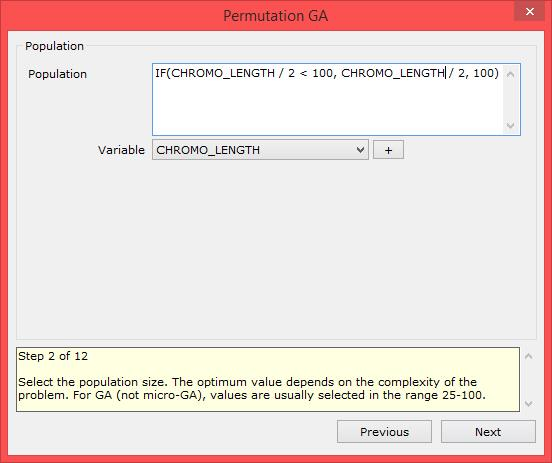Enter the population size for the GA. Usually the optimum value depends on the complexity and the nature of the problem. A mathematical expression may also be used.

• Example 1: entering '50' sets the population size to 50 individuals.
• Example 2: entering 'IF(CHROMO_LENGTH / 2 < 100, CHROMO_LENGTH / 2, 100)' sets the population size to the chromosome length divided by two if the result is less than 100, or 100 otherwise.

The variables that may be used in the expression are:

• CHROMO_LENGTH: the chromosome length (equal to the number of design variables).

#### Step 3Choose the selection method. The method can be either tournament selection or biased roulette wheel. Usually, tournament selection with pool size equal to 2 and probability equal to 1 is adequate. The roulette wheel method requires the definition of a fitness function, based on the objective function. The evaluation of the fitness can be performed automatically, or based on custom code. In the latter case, the variables that can be used are the following:

• MIN_OV: the minimum objective value within the members of the population.
• MAX_OV: the maximum objective value within the members of the population.
• AVER_OV: the average objective value within the members of the population.
• BEST_OV: the best objective value within the members of the population.
• WORST_OV: the worst objective value within the members of the population.
• OV: the objective value of the current individual for which the fitness is being evaluated.
• TARGET: the target objective value for target - problems.

The automatic evaluation is based on the following code:

• '(OV - MIN_OV) / AVER_OV' for maximization problems,
• '(MAX_OV + MIN_OV - OV) / AVER_OV' for minimization problems,
• '(ABS(MAX_OV - TARGET) + ABS(MIN_OV - TARGET) - ABS(OV - TARGET) / ABS(AVER_OV - TARGET)' for target-value problems.

Based on the above, a first level of normalization is achieved with the division by the average objective value.

#### Step 4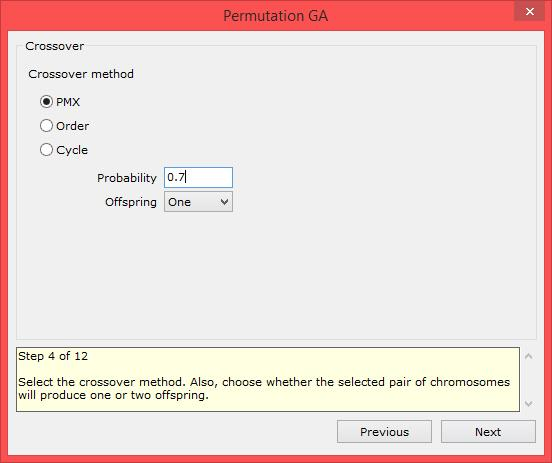Select the crossover method. Usually PMX or Order crossover is used for adjacency problems. Cycle crossover is used for problems where the absolute position in the string is important. Also, choose whether the selected pair of chromosomes will produce one or two offspring. The crossover methods are described in detail in .

#### Step 5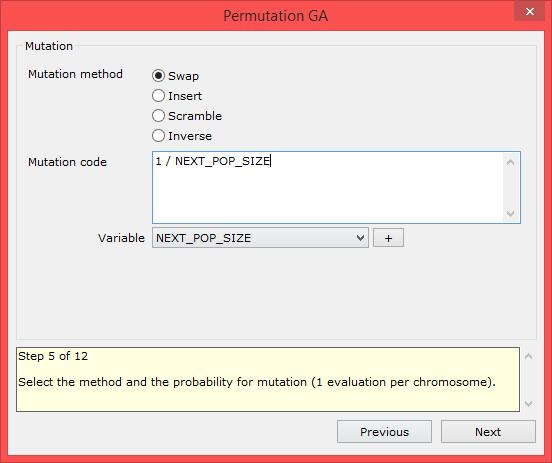Select the method and probability for mutation. The methods available are Swap, Insert, Scramble and Inverse. These are explained in detail in .

• Example 1: the mutation code is '1 / NEXT_POP_SIZE'. Note that the random number generator is invoked NEXT_POP_SIZE times, therefore one chromosome per generation is expected to be mutated, on average.

#### Step 6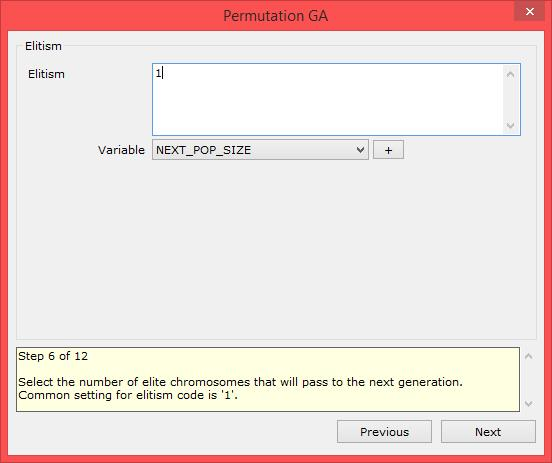Select the number of elite chromosomes that will pass to the next generation. Common setting for elitism code is '1', i.e. the single best individual is transferred to the next generation.

#### Step 7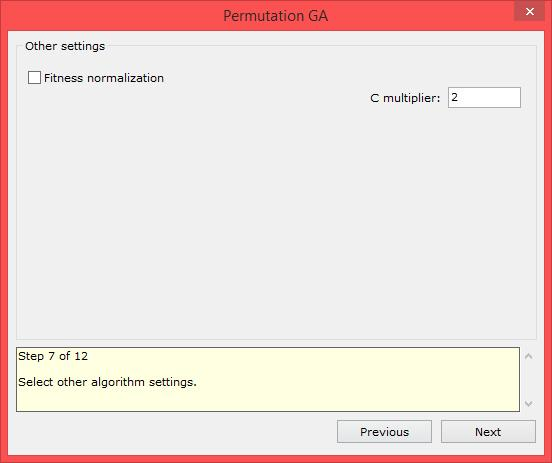Select other algorithm settings. The options are the following:

• Fitness normalization: Choose whether a fitness normalization method is applied, using the specified c-coefficient. The normalization is not important if you use rank selection schemes, such as tournament selection.

#### Step 8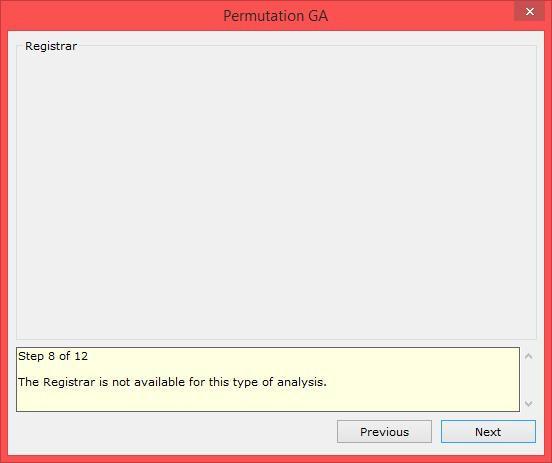At present, the Registrar operator is not available with GAPerm.

#### Step 9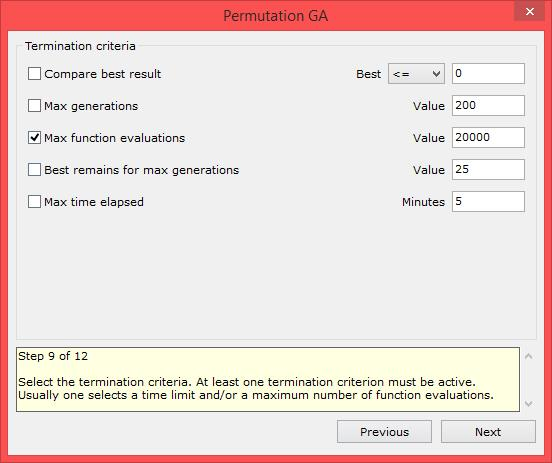Select the termination criteria. At least one termination criterion must be active. Usually one selects a maximum number of function evaluations and/or a time limit.

#### Step 10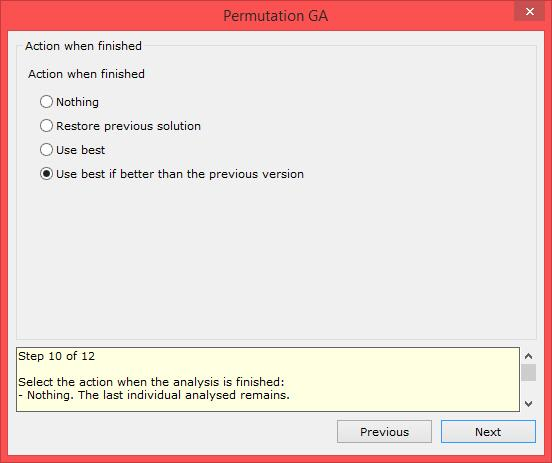Select the action when the analysis is finished:

• Nothing: The last individual analysed remains.
• Restore previous solution: The solution that was visible before the analysis is restored.
• Use best: The best solution that was discovered during the analysis is applied.
• Use best if better than the previous version (recommended): The best solution that was discovered during the analysis is applied only if it is better than the previous solution. In the opposite case the previous solution is restored.

#### Step 11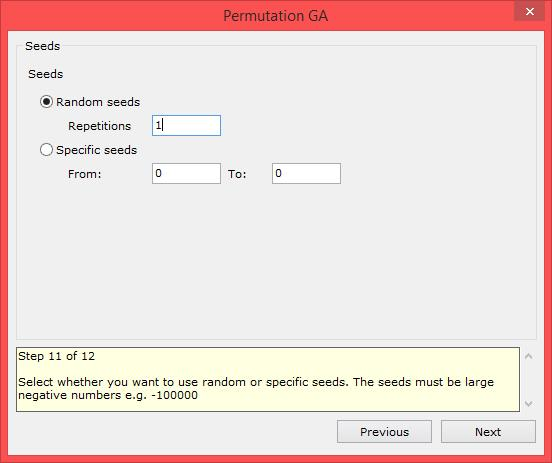Select whether you want to use random or specific seeds for the random number generator. The seeds must be large negative numbers e.g. -100000. Usually a number of analyses with random seeds is performed. The filename of the log is the seed used in the specific analysis.

#### Step 12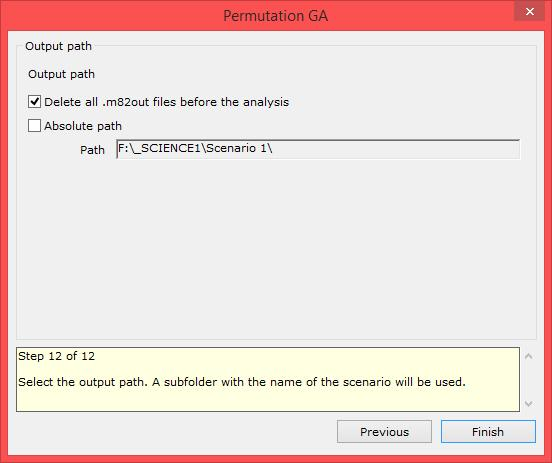Select the output path. A subfolder with the name of the scenario will be used. Usually a relative path is used i.e. the absolute path check box is not checked. This results in the output logs being saved in the same path as the project file.